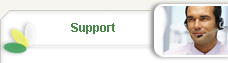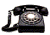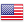+ 1-888-787-5890+ 1-302-351-4405

# Essay/Term paper: Rates of reaction

## Essay, term paper, research paper:  Science Research Papers

Free essays available online are good but they will not follow the guidelines of your particular writing assignment. If you need a custom term paper on Science Research Papers: Rates Of Reaction, you can hire a professional writer here to write you a high quality authentic essay. While free essays can be traced by Turnitin (plagiarism detection program), our custom written essays will pass any plagiarism test. Our writing service will save you time and grade.

Rates of Reaction

BACKGROUND INFORMATION

What affects the rate of reaction? 1) The surface area of the magnesium. 2)
The temperature of the reaction. 3) Concentration of the hydrochloric acid. 4)
Presence of a catalyst.

In the experiment we use hydrochloric acid which reacts with the magnesium to
form magnesium chloride. The hydrogen ions give hydrochloric acid its acidic
properties, so that all solutions of hydrogen chloride and water have a sour
taste; corrode active metals, forming metal chlorides and hydrogen; turn litmus
red; neutralise alkalis; and react with salts of weak acids, forming chlorides
and the weak acids.

Magnesium, symbol Mg, silvery white metallic element that is relatively
unreactive. In group 2 (or IIa) of the periodic table, magnesium is one of the
alkaline earth metals. The atomic number of magnesium is 12.

Magnesium(s) + Hydrochloric acid(aq) = Magnesium Chloride(aq) + Hydrogen(g)
Mg + 2HCl = MgCl2
+ H2

In the reaction when the magnesium hits the acid when dropped in, it fisses and
then disappears giving of hydrogen as it fisses and it leaves behind a solution
of hydrogen chloride.
The activation energy of a particle is increased with heat. The particles
which have to have the activation energy are those particles which are moving,
in the case of magnesium and hydrochloric acid, it is the hydrochloric acid
particles which have to have the activation energy because they are the ones
that are moving and bombarding the magnesium particles to produce magnesium
chloride.

The rate at which all reactions happen are different. An example of a fast
reaction is an explosion, and an example of a slow reaction is rusting. In any
reaction, reactants chemical reactions® products.

We can measure reactions in two ways:

1) Continuous:- Start the experiment and watch it happen; you can use a
computer "logging" system to monitor it. I.e. Watching a colour fade or
increase.

2) Discontinuous:- Do the experiments and take readings/ samples from the
experiment at different times, then analyse the readings/samples to see how many
reactants and products are used up/ produced.

Reaction rate = amount of reactant used up
time taken

If the amount used up is the same each time then the only thing that changes is
the time taken.

so, reaction rate µ 1
time taken.
rate = K
time taken.

Where K is the constant for the reaction.

For particles to react:-

a) They have to collide with each other. b) They need a certain amount of
energy to break down the bonds of the particles and form new ones. This energy
is called the "Activation Energy" or Ea.

When we increase the temperature we give the particles more energy which:

1) Makes them move faster which In turn makes them collide with each other more
often.

2) Increases the average amount of energy particles have so more particles have
the "activation energy"

Both of these changes make the rate of reaction go up so we see a decrease in
the amount of time taken for the reaction and an increase in time taken.
= 1

Time taken reflects the rate of reaction.

Because temperature has an effect on both the speeds at which the particles
react and the activation energy they have a greater effect on the rate of
reaction than other changes.

A change in concentration is a change in the number of particles in a given
volume.

If we increase the volume:-a) The particles are more crowded so they collide
more often.

b) Although the average amount of energy possessed by a particle does not
change, there are more particles with each amount of energy;- more particles
with the activation energy.

a) is a major effect which effects the rate, but b) is a minor effect which
effects the rate very slightly.

In this experiment we are not concerned with whether the reaction is
exothermic or endothermic because we are concerned with the activation energy
needed to start and continue the reaction.

PREDICTIONS

I predict that as we increase the temperature the rate of reaction will
increase.
If we increase the temperature by 100C the rate of reaction will double.

I predict that if we increase the concentration of the acid the reaction rate
will increase.
If the concentration of the acid doubles, the rate of the reaction will also
double.

Reaction Rate and Temperature.
The collision theory describes how the rate of reaction increases as the
temperature increases. This theory states that as the temperature rises, more
energy is given to the particles so their speed increases, this increases the
number of collisions per unit of time. This increase in collisions increases
the rate of reaction.
The collision theory explains how the rate of reaction increases, but it does
not explain by how much or by how fast the rate increases. The Kinetic energy of
a particle is proportional to its absolute (Kelvin) temperature.
1/2 mv 2µ T
But the mass of the particles remains constant so we can eliminate that part of
the equation so;
Þ V2µT
Therefore we can fit this into a formula:
V21/V22 = T1/T2
If we substitute the temperature into the formula we can work out the average
speed of the formula:
V21/V22 = 310/300
V 1 = Ö310/300V 2
= Ö1.033V2
= 1.016V2

However if we look at this it is only 1.016 times greater than the speed at
300K, in other words we can see that it has only increased by 1.6%.
The frequency of the collisions depends on the speed of the particles, this
simple collision theory only accounts for the 1.6% increase in the rate, but in
practice the reaction rate roughly doubles in a 10K rise, so this simple theory
cannot account for an 100% increase in the reaction rate.
During a chemical reaction the particles have to collide with enough energy to
first break the bonds and then to form the new bonds and the rearranged
electrons, so it is "safe" to assume that some of the particles do not have
enough energy to react when they collide.
The minimum amount of energy that is needed to break down the bonds is called
the activation energy (EA). If the activation energy is high only a small
amount of particles will have enough energy to react so the reaction rate would
be very small, however, if the activation energy is very low the number of
particles with that amount of energy will be high so the reaction rate would be
higher. An example of a low EA would be in explosives when they need only a
small input of energy to start their exceedingly exothermic reactions.
In gases the energy of the particles is mainly kinetic, however in a solid of a
given mass this amount of energy is determined by their velocities.

This graph below shows how the energies of particles are distributed.

This graph is basically a histogram showing the number of particles with that
amount of energy. The area underneath the curve is proportional to the total
number of particles. The number of particles with > EA is proportional to the
total area underneath the curve.
The fraction of particles with > EA is given by the ratio:

Crosshatched area under the curve
total area under curve

Using the probability theory and the kinetic theory of gases, equations were
derived for the distribution of kinetic energy amongst particles. From these
equations the fractions of particles with an energy > EA J mole-1 is represented
by the equation: e -Ea/RT where R= the gas constant (8.3 J K-1 mole -1)
T= absolute temperature.
This suggests that at a given temperature, T,
The reaction rate µ e -Ea/RT
If we use k as the rate constant, as a measure of the reaction rate we can put
this into the equation also.
kµ e -Ea/RT
Þ k= A e -Ea/RT
The last expression is called the Arrhenius equation because it was developed
by Srante Arrhenius in 1889. In this equation A can be determined by the total
numbers of collisions per unit time and the orientation of the molecules when
the collide, whilst e -Ea/RT is determined by the fraction of molecules with
sufficient amounts of energy to react.

Putting the probability theory and the kinetic theory together this now gives
us a statement which accounts for the 100% increase in the rate of reaction in a
10K rise.

Reaction Rate and Concentration.

The reaction rate increases when the concentration of the acid increases
because:

If you increase the concentration of the acid you are introducing more particles
into the reaction which will in turn produce a faster reaction because there
will be more collisions between the particles which is what increases the
reaction rate.

METHOD.

To get the amount of magnesium and the amount of hydrochloric acid to use in
the reaction, we have to use an excess of acid so that all of the magnesium
disappears.
Mg + 2HCl = MgCl2
+ H2
1 mole 2 moles 1 mole
1 mole

So, we can say that one mole of magnesium reacts with 2 moles of hydrochloric
acid.
If we use 1 mole of magnesium and 2 moles of hydrochloric acid we will get a
huge amount of gas, too much for us to measure. We would get 24,000 cm3 of
hydrogen produced where we only want 100 cm3 of hydrogen produced. So to get
the formula for the amount of moles that we have to use the formula:
Moles = mass of sample 100 =0.004 moles.
volume with 1 mole 24,000

To get the maximum mass we can use:
Mass = moles x RAM.
= 0.004 x 24
= 0.0096g
So, this is the maximum amount of magnesium we can use. To the nearest 0.01 of
a gram = 0.01. This is the maximum amount of magnesium we can use.
Because the reaction reacts one mole of magnesium to two moles of hydrochloric
acid we have to make sure that even with the lowest concentration of acid we
still have an excess of acid.
The acid that we were using was 2 moles per dm2 which means that it is 0.2
moles per 100 cm2 of acid.
We need to make the reaction work to have double the amount of magnesium. The
maximum number of moles that the magnesium needed was 0.004 moles so the amount
of acid that we needed was double that so that equals 0.008 moles. As you can
see from the table below we have the acid in excess throughout the experiment.
Amount of HCl (cm3) Amount of H2O (cm3) Moles of acid. 100 0
0.2 75 25 0.15 50 50 0.1 25 75 0.05 The reason why we
used 0.01g of magnesium was because it was therefore easy to measure because
there was not too much, or too little. Therefore we had no problem with too
much gas.

Apparatus
This is the apparatus we used to measure the amount of H2 that was produced in
the reactions. We measured the amount of gas that was given of every two
seconds to get a good set of results. We used this apparatus with the reaction
changing the concentration, and then the temperature. To accurately measure the
amount of gas given of we used a pen and marked on the gas syringe at the time
intervals.
This is the apparatus we used to measure how long it took for the magnesium to
totally disappear. We used this apparatus in both of the experiments, changing
the temperature and the concentration of the acid to water.

Temperature.
When we did the experiment changing temperature we used both of the sets of
apparatus. To get a fair reaction we had to keep the amount of magnesium the
same and the concentration of the acid. In the experiment we used 0.1g of
magnesium and the concentration of the acid was 50cm3 of acid to 50cm3 of water.
This is because if we used 100cm3 of acid the reaction would be too fast. Still
we had an excess amount of acid, so one mole of magnesium can react with two
moles of HCl. Concentration.
When we did the reaction changing the concentration we changed the
concentration until we had just enough for 1 mole of magnesium to react with two
of HCl. To get a fair reaction we had to keep the amount of magnesium the same
and the temperature. We used 0.1g of magnesium.

RESULTS

Temperature

From this graph you can see that if we do increase the temperature the rate of
reaction also increases, but it does not show that if you increase the
temperature the rate of reaction doubles.

This graph shows that there is an increase in the rate of reaction as the
temperature increases. This shows a curve, mainly because our results were
inaccurate in a number of ways. This is because the concentration is changed
during the experiment because at high temperatures the acid around the magnesium
is diluted. If this experiment was accurate it would be also a curve but if you
made it into 1/time the result would be a straight line showing a clear
relationship.

Even though I changed it to 1/time it still does not show a clear relationship
because of the factors mentioned in the conclusion. Concentration

This graph shows an increase in the amount of gas given off and the speed at
which it is given off. This graph also does not show the rate increase, it just
shows how it increases with a change in concentration.

This graph shows that if you increase the concentration of the molar solution of
the acid the time in which the Mg takes to disappear becomes a lot slower. This
does not show the rate at which this happens, the graph of rate vs. conc. would
show a straight line.

This shows a straight line, thus proving that there is a relationship between
the time it takes the magnesium to disappear and the concentration of the acid.
If we take a gradient of it, it would show the rate at which the reaction was
happening.

Because this shows a straight line we can say that it is a second order
reaction.

This graph shows a nearly straight line which shows that there is a
relationship between the temperature and the rate of reaction, as the
gradient shows the rate of reaction. If you look at this graph it comes out to
show that if you increase the temperature by 100C the gradient of the line is
doubled. This shows that rate µ temp.

This graph shows that if you increase the molar concentration of the acid, you
will increase the rate of reaction. From this you can see from the gradient,
that if you double the molar concentration of the acid the rate of reaction will
double because the gradient is a way of showing the rate of reaction.
If you compare the quantitative observations to see which the faster reaction
is you can see that after 10 seconds: Temp. 2 10 20 30
40 50 Amount of H2 produced after 10s 7.5 16 25 54
57 83
Even though there is a greater increase in the amount of H2 given off in each
of the different reactions you can see that there is a change in the amount
given off, but between the temperatures 30 and 400C there is not much of a
change, this could be because of our human error, there should be a big change
in the amount given off. Molar conc. 0.5 1 1.5 2 Amount of H2
produced after 10s 6 25 60 90 This table shows a nice
spread of results throughout the range of concentration. It clearly shows that
the reaction is at different stages so is therefore producing different amounts
of H2. This shows also that the reaction is affected by the concentration of
the acid.

CONCLUSIONS

I conclude that if you increase the temperature by 10oC the rate of reaction
would double, this is because of using the kinetic theory and the probability
theory. Even though our results did not accurately prove this, the theory that
backs it up is sufficient. the kinetic theory explains that if you provide the
particles with a greater amount of kinetic energy they will collide more often,
therefore there will be a greater amount of collisions per unit time. The
probability theory explains that there is only a number of particles within the
reaction with the amount of Ea to react, so if you increase the amount of
kinetic energy there will be more particles with that amount of Ea to react, so
this will also increase the reaction rate.
If you double the concentration of the acid the reaction rate would also double,
this is because there are more particles in the solution which would increase
the likelihood that they would hit the magnesium so the reaction rate would
increase. The graph gives us a good device to prove that if you double the
concentration the rate would also double. If you increase the number of
particles in the solution it is more likely that they will collide more often.
There should be more H2 given off if we compare it across the range of
temperatures because the reaction is going quicker and so more H2 is given off
in that amount of time.
There is more H2 given off if you compare it to the range of concentrations
that you are using, this shows that the reaction is at different stages and so
is therefore producing different amounts of H2.
Also our results were not accurate but this could be because of a number of
reasons.
There our many reasons why our results did not prove this point accurately. -
At high temperatures the acid around the magnesium starts to starts to dilute
quickly, so if you do not swirl the reaction the magnesium would be reacting
with the acid at a lower concentration which would alter the results. - Heating
the acid might allow H Cl to be given off, therefore also making the acid more
dilute which would also affect the results. - When the reaction takes place
bubbles of H2 are given off which might stay around the magnesium which
therefore reduces the surface area of the magnesium and so the acid can not
react properly with it so this affects the results.

To get more accurate results, we could have heated the acid to a lower
temperature to stop a large amount of H Cl being given off. The other main
thing that could have helped us to get more accurate results is we cold have
swirled the reaction throughout it to stop the diluting of the acid and the
bubbles of H2 being given off.

If I had time I could have done the reactions a few more times to get a better
set of results. This would have helped my graphs to show better readings.

## Other sample model essays:

Chemistry / Robert Boyle
Robert Boyle Robert Boyle is considered both the founder of modern chemistry and the greatest English scientist to live during the first thirty years of the existence of the Royal Society....
The Role Catalysts In Chemical Reactions, Their Importance In Industry, Problems and New Developments OXFORD AND CAMBRIDGE SCHOOLS EXAMINATION BOARD. General Certificate Examination - Advan...
The Safety Inspector Mr. Redos, I am an inspector for the OSHA. I have noticed the following safety objects missing in the room F 203, chemistry room. These are sprinklers, a drain, and ...
Biography Term Papers / Andy Warhol
Andy Warhol Never before have I encountered more intriguing works of art than those done by Andy Warhol. I have been curious about his life ever since I saw his work in Milwaukee. I saw h...
Science Fair Project: Melting Ice Materials: - 3 aluminum baking pans - water to fill each pan - 1/2 cup of salt - 1/2 cup of rubbing alcohol - 1/2 cup of sand - watch - large freeze...
Creative Writing: The Fossilized Story of Mr. Allosaurus As the mud starts to surround me I am no longer able to breathe. I catch my last gasp of air and feel a few last raindrops fall on ...
The Sequence of Chemical Reactions Drew Selfridge Dave Allen, Lab partner Instructor Yang February 11, 1997 INTRODUCTION This experiment was to recover the most amount of copper after i...
Chemistry / Chemical Engineer
Chemical Engineer The chemical engineer is an invaluable link between scientific principles and manufacturing realities. It involves the use of chemical, physical, and engineering princip...
Science Research Papers / Silicon
Silicon Silicon is the raw material most often used in integrated circuit (IC) fabrication. It is the second most abundant substance on the earth. It is extracted from rocks and comm...
Science Research Papers / Technetium
Technetium Nalin Balan NUCL 200 Paper due 02/07/97 Atomic Number: 43 Atomic Symbol: Tc Atomic Weight: (97) Electron Configuration: -18-13-2 History, Pro...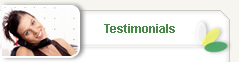Experience with Dream Essay - Reliable and great customer service. Quality of work - High quality of work.
Browns Mills, New Jersey, United States
Dream Essay - Very reliable and great customer service. Encourage other to try their service. Writer 91463 - Provided a well written Annotated Bibliography with great deal of detail per the rubric.
Browns Mills, New Jersey, United States
it is always perfect
Frederick, Maryland, United States
The experience with Dream Essay is stress free. Service is excellent and forms various forms of communication all help with customer service. Dream Essay is customer oriented. Writer 17663 is absolutely excellent. This writer provides the highest quality of work possible.
Browns Mills, New Jersey, United States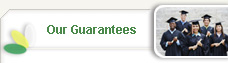Only competent & proven writers
Original writing — no plagiarism
Our papers are never resold or reused, period
Satisfaction guarantee — free unlimited revisions
Client-friendly money back guarantee
Total confidentiality & privacy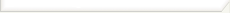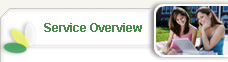Live Chat & 24/7 customer support
All difficulty levels
12pt Times New Roman font, double spaced, 1 inch margins
The fastest turnaround in the industry
Fully documented research — free bibliography guaranteed